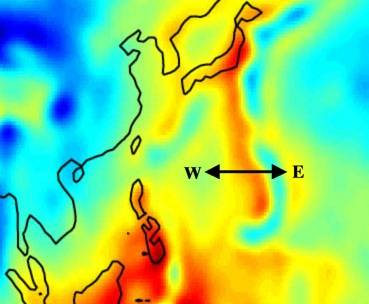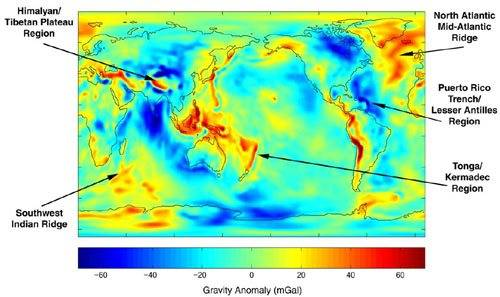# Gravitational acceleration at Mariana Trench

• new_r
If you want to measure g as precisely as possible, you need to take into account the centrifugal acceleration. This acceleration is due to the Earth's rotation, and it affects the gravity field at a given location.f

#### new_r

Maybe someone can help with this question:
How to calculate as possible precisely gravitational acceleration (g) at Mariana Trench?

Some questions:
- Do you want the answer at sea surface, or at the bottom of the trench?
- Do you know what spherical harmonics are?

let the value to be calculated is equal to 'g'
so weight of that body is precisely the force due to Earth's attraction:
or, mg=GMm/(r*r)
here, m is mass of the object and M = mass of that part of Earth enclosed by a sphere having radius same as distance of Earth's center from mariana trench(= r)
calculate and please let me know the percentage decrease in the value of g.

mg=GMm/(r*r)
You are assuming spherical gravity here. The OP asked for a calculation as precise as possible.

... precisely gravitational acceleration (g) at Mariana Trench?
you can easily find it yourself, if you choose a particular place: the deepest spot [discovered in 2009] is 10,971 m closer to Earth's center than see level, if you are looking for max g, [as Earth is not a perfect sphere] some parts of the Arctic Ocean are 13,000 m closer then Marianas.
you simply ought to find out what is g at the latitude you choose.

Last edited:
I found a plot of density and g here:
http://members.arstechnica.com/x/bravehamster/gravity.GIF [Broken]

It looks at very beginning (red line from right to left) g arise linearly.
Something like dg/dr = k
But it is difficult to estimate from this plot what this k is.

If the beginning of this plot is really almost linear
for me would be enough to know mentioned coefficient k.
But this k likely also depends on density (we have water in this case).

g on a surface of the Earth likely can be find from Goce data
http://www.bbc.co.uk/news/science-environment-12911806

Last edited by a moderator:
I found a plot of density and g here:
http://members.arstechnica.com/x/bravehamster/gravity.GIF [Broken]
That's the Preliminary Reference Earth Model, see http://geophysics.ou.edu/solid_earth/prem.html [Broken]. That model is not going to help you much if you really do want a precise number.

g on a surface of the Earth likely can be find from Goce data
http://www.bbc.co.uk/news/science-environment-12911806
The GOCE data are still preliminary, but you can find some space-only spherical harmonics models based on GOCE, GRACE, CHAMP, and SLR. The GOCO02S model coefficients are at http://ftp.tugraz.at/pub/goco/GOCO02S/GOCO02S.gfc. To use these, you are going to have to find the geoid at your target location. That alone is going to be a bit tough; those deep sea trenches are places where the geoid height varies a lot. You can find software to help with this process on the web. Now that you have the geoid you can assume that mean sea level is the geoid. So now you can get g by applying the spherical harmonics. Since this is a space-only solution, you are going to have to add in the centrifugal acceleration at that point. (g includes acceleration due to gravity and centrifugal acceleration).

Now it's going to get tough if you really do want g at the bottom of the trench. As a first cut, make a negative free air correction and a negative double Bougeur correction assuming a density of sea water. However, the Bougeur correction assumes an infinite flat plate of constant density. This is a reasonable assumption for terrain whose elevation changes gradually. It is not a such good assumption for sharp mountains and deep trenches. Particularly so for those deep trenches.

Last edited by a moderator:
you simply ought to find out what is g at the latitude you choose.
you are going to have to add in the centrifugal acceleration at that point.[PLAIN]http://www.uklv.info/g.php[/QUOTE] [Broken]
if one gets precise value of g at that place, isn't it inclusive of all factors?

Last edited by a moderator:
As I see here may be too much factors to get real numbers.
But let's consider we want practically to measure g by immersing some probe.
Lets say this probe has construction possibilities to come out later.
What types of g-meters inside this probe would be able to measure most accurate results?

you are going to have to add in the centrifugal acceleration at that point.
When I said "add" I mean vector sum. Gravitation is directed inward, more or less toward the center of the Earth, centrifugal force is directed outward, away from the Earth's rotation axis. In physical geodesy, gravity and gravitation are two different things. Gravitation is the inward acceleration described by Newton's law of gravitation. Gravity is the vector sum of the gravitational and centrifugal accelerations.

The reason for including centrifugal acceleration is that the value of g at some location on the surface of the Earth is defined as the acceleration of a falling object falling at that point as observed at that point. The observation is made from the perspective of a rotating frame of reference. So of course fictitious forces come into play.

__________________________________________________

An alternative to using spherical harmonics coefficients is to use a gravity anomaly map. This map of the Izu-Bonin-Mariana arc region is based on GRACE data rather than GOCE, so not quite so much resolution as GOCE should yield:The Mariana Trench is that bluish arc at the east end of the west-east arrow. The blue represents a negative gravity anomaly. One can also see positive gravity anomalies in the forearc to the east (yellow) and backarc to the west (red). The color scale is the same as that on this map:The gravity anomaly in these maps is the deviation in mGals (10-5 m/s2; 1 mGal = one milligalileo, and a galileo is one centimeter per second squared) from that due to an ideal oblate spheroid model of the Earth,

$$g_{\phi} = 9.780327(1+0.0053024\sin^2\phi - 0.0000058\sin^2 2\phi)\;\text{m}/\text{s}^2$$

Last edited by a moderator:
1) As I see here may be too much factors to get real numbers.
2) But let's consider we want practically to measure g by immersing some probe.
Lets say this probe has construction possibilities to come out later.
1)What types of g-meters inside this probe would be able to measure most accurate results?
1) what is the order of precision you would like to achieve?, if precise g at sea level is available, ordinary formula won't satisfy you?
2) how do you imagine this probe? a [hollow] steel sphere on the bottom of the trench? could this be more accurate in your opinion?

Last edited:
As I see here may be too much factors to get real numbers.
But let's consider we want practically to measure g by immersing some probe.
Lets say this probe has construction possibilities to come out later.
What types of g-meters inside this probe would be able to measure most accurate results?
MIMS accelerometers with sensitivities on the order of a hundred milligals are a dime a dozen; you might well have one in your cellphone. Custom inertial measurement units with sensitivities on the order of a milligal are used in airplanes, spacecraft , ships, and submarines. Superconducting gravimeters with sensitivities on the order of a nanogal have been built, but you are not going to be able to put such a device in your hypothetical probe.

Maybe someone can help with this question:
How to calculate as possible precisely gravitational acceleration (g) at Mariana Trench?

If you want it as precise as possible you must send down an instrument, measure it directly. The degree of precision you obtain depends upon your resources available to investigate and evaluate the reliability of suppliers of gravitometric instuments.

Not the answer you wanted to hear was it? How do you know a kilogram mass standard is really a kilogram?

Than you for useful information.

This is not so easy to estimate necessary precision.
Let I just write final purpose of these questions:
There is physical value Φ – gravitational potential
http://en.wikipedia.org/wiki/Gravitational_potential

Lets introduce another similar physical value.
Lets mark it for now like Θ and describe it like so:
Θ = g*r
where g is total gravitational acceleration and
r is radius of curvature of gravitational force.
This r can be found like so.
(if link will not work just copy paste it to your browser)
Lets consider some point in space (black dot)
Then let's take some very close point (gray point) and find where vectors of gravitational
forces intersects (red point)
Now we have, r is distance between black and red points.

Φ almost equals to Θ outside our planet.
But inside they may differ a lot.
For example at the center of the planet Θ will be almost 0
The reason/target of previous questions was how to calculate or measure the difference
between Φ and Θ at Mariana Trench or at some other places where it is measurable difference.

Or maybe better I need to look for differences at some places in space?
Maybe at the points where Earth's and Sun's gravitational forces becomes equivalent?

Last edited:
What are you rambling on about?

What are you rambling on about?

I think that, not for the first time, the deluge of answers just didn't match such a vague original question.
They just wind us up and off we go in random directions, answering a range of alternative questions that we made up in our own heads.
(More rambling?)

I just was tying to explained the target of the initial question.
From this point you may better see/estimate the accuracy I need.
(Because there was question about necessary accuracy.)

I just need some realistic points for realistic experiments where Θ and Φ differs maximally.
The target of experiments is to detect who is really response for gravitational time dilation and gravitational shift of photon's frequency Φ or Θ.
For that I need the data how to calculate gravitational forces inside deep places.
Your information was very useful, thank you,
but if there are some additional minds I would be thankful.

By the way similar experiment was proposed by people from Los Alamos National Laboratory 20 Years ago http://www.sciencedirect.com/science/article/pii/037596019190554L
But it looks such experiment still was not done.

I think that, not for the first time, the deluge of answers just didn't match such a vague original question.

I wonder if sometimes people deliberately ask vague nonsense questions, or questions based on confused vapor like the last couple posts, just to sit back and watch the posts fly. :rofl:

Perhaps we should try doing the same thing.